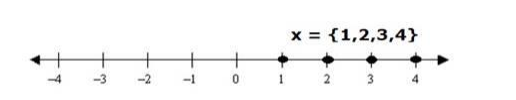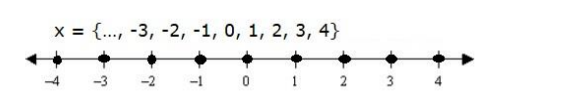# Solve each of the following in equations and represent the solution set on

Question:

Solve each of the following in equations and represent the solution set on the number line.

$6 x \leq 25$, where (i) $x \in N$, (ii) $x \in Z$.

Solution:

(i) $6 x \leq 25, x \in N$

Dividing both the sides by 6 in the above equation,

$\frac{6 x}{6} \leq \frac{25}{6}$

$x \leq \frac{25}{6}$

x ≤ 4.166

Since x is a natural number, therefore the value of x can be less than or equal to 4

Therefore, x = {1,2,3,4}(ii) $6 x \leq 25, x \in Z$

Dividing both the sides by 6 in the above equation,

$\frac{6 x}{6} \leq \frac{25}{6}$

$x \leq \frac{25}{6}$

x ≤ 4.166

Since x is an integer so the possible values of x can be:

$x=\{\ldots,-3,-2,-1,0,1,2,3,4\}$